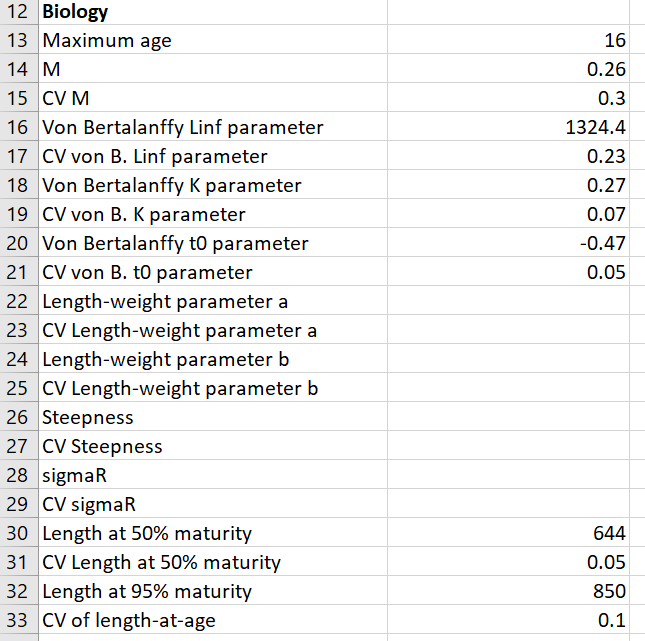## Biology

The next section contains mean and uncertainty values for the biological parameters of the species. Leave any entry blank if the parameter is unknown.The Biology section in the Data Documentation file is indicated with “## Biology”. Enter any relevant supporting information for the information in the Biology section of the input file here.

Ideally a short paragraph or two with supporting information for each type of biological data entered in the Data Input file.

Use in-text citations where possible and include references under the “## Reference List” section.

Do not delete or modify the “## Biology” heading.

### Maximum age

Numeric entry. The maximum age of the species. The catch-at-age data entries must match this value (see the [Catch-at-Age] section for more details).

The cobia example has a maximum age of 16.

### M and CV M

Numeric entries. A point estimate for the (adult) natural mortality rate (M) and a coefficient of variation (CV) associated with this estimate (assuming a log-normal distribution).

The cobia example has an estimate of M of 0.26 and an associated CV of 0.3.

### von Bertalanffy Linf parameter and CV

Numeric entries. The estimated mean asymptotic length from a fitted von Bertalanffy growth model and the associated CV.

The units of the Linf parameter are not important, but all length parameters and data (e.g., length-at-maturity and catch-at-length) must be in the same units.

The cobia example has an estimate of Linf of 1324.4 and an associated CV of 0.23.

### von Bertalanffy K parameter and CV

Numeric entries. The estimated von Bertalanffy growth parameter (K) and the associated CV.

The K parameter must be in the same units as M, usually $\text{year}^{-1}$.

The cobia example has an estimate of K of 0.27 and an associated CV of 0.07.

### von Bertalanffy t0 parameter and CV

Numeric entries. The estimated age when mean length is zero (t0) and the associated CV.

The t0 parameter must be in the same units as “Maximum age” (usually years).

The cobia example has an estimate of t0 of -0.47 and an associated CV of 0.05.

### Length-weight parameters

Numeric entries. Estimates of the a and b parameters (and associated CVs) from a fitted length-weight model of the form:

$$W=aL^b$$

This data is not available for the example cobia data.

### Recruitment parameters

Numeric entries. Mean estimates and associated CVs.

The steepness parameter is the expected fraction of virgin recruitment when the spawning biomass has been reduced to 20% of the unfished level. This is an important parameter for determining the productivity of the stock, especially at low levels of spawning biomass. However, the parameter is difficult to estimate and not well known for many species.

The sigmaR parameter describes the variance around the expected stock-recruitment relationship.

This data is not available for the example cobia data.

### Length-at-Maturity parameters

Numeric entries. Mean estimates and associated CVs.

The Length at 50% maturity and Length at 95% maturity parameters are estimated by fitting a logistic model to maturity-at-length data. The parameters refer to the expected length where 50% and 95% respectively of the population are mature. The CV of length at 95% maturity is assumed to be the same as the CV of length at 50% maturity.

The example cobia data has estimates of the length at 50% and 95% maturity of 644 and 850 mm respectively, and a CV of 0.05.

### Variability of length-at-age

Numeric entry. The expected variability of length-at-age; that is, the distribution of length-at-age around the mean growth curve described by the von Bertalanffy growth model.

The example cobia data assumed a coefficient of variability of length-at-age of 0.1.